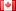Ask Your Question

# Revision history [back]

Hello,

First of all, your code works! The problem is that you need to specify a value to the variables a and b. The best way should be to put all that in a function as in the following

def euclide(a,b):
r=a%b
print (a,b,r)
while r != 0:
a=b; b=r
r=a%b
print (a,b,r)


Then you can do

sage: eudlide(12,5)
(12, 5, 2)
(5, 2, 1)
(2, 1, 0)


To use an external file, it depends on how do you use Sage : in a console or with the notebook ?

Vincent2 fixed typofidbc2288 ●4 ●23 ●54

Hello,

First of all, your code works! The problem is that you need to specify a value to the variables a and b. The best way should be to put all that in a function as in the following

def euclide(a,b):
r=a%b
print (a,b,r)
while r != 0:
a=b; b=r
r=a%b
print (a,b,r)


Then you can do

sage: eudlide(12,5)
euclide(12,5)
(12, 5, 2)
(5, 2, 1)
(2, 1, 0)


To use an external file, it depends on how do you use Sage : in a console or with the notebook ?

Vincent